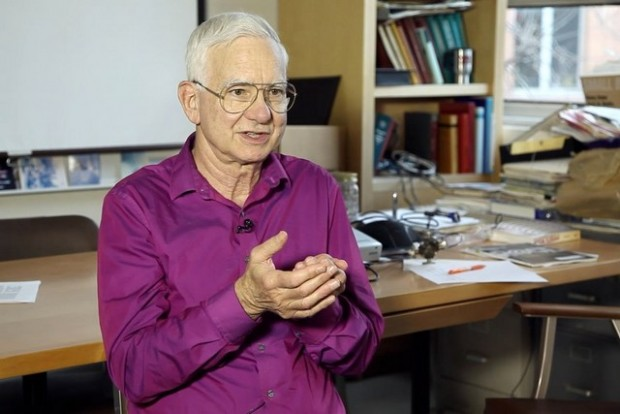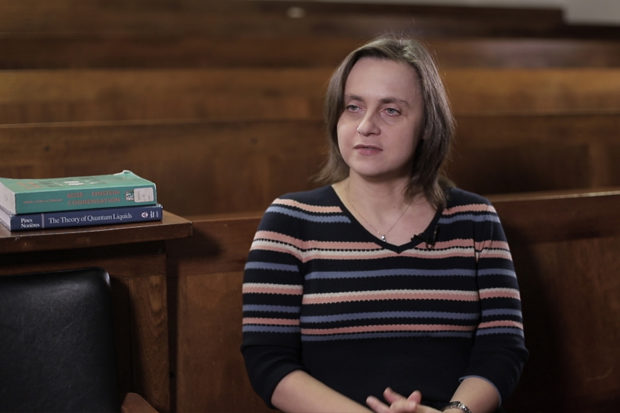# Maxwell’s Equations

## Physicist Martin McCall on electromagnetic induction, Ampere’s experiments, and magnetic monopoles

### videos | December 12, 2016

The video is a part of the project British Scientists produced in collaboration between Serious Science and the British Council.
Maxwell’s equations a set of four equations that physicists learn about in their undergraduate studies are four fundamental equations that describe everything that we know about electric fields and magnetic fields and ultimately leads us to that the classical theory that describes how light behaves. The history towards Maxwell’s equations is quite long and the equations themselves were first published in 1961.

But the path towards Maxwell’s equations includes many scientists, many steps. And we can break those down into different parts. Many of us are familiar with the idea that if you have an electric charge and we have another electric charge just here that they are either attracted to each other or repelled from each other according to the positive or negative. And in fact, this attraction or repulsion follows an inverse square law similar to the law of gravitation. And this is called Coulomb’s law, it was discovered in 1874. And so that’s the first equation, that’s the first part of the jigsaw that makes up Maxwell’s equations. This idea of the attraction and repulsion of charges.Physicist David Pritchard on the efficiency of cold atom experiments, Bose-Einstein condensation, and the use of high-temperature superconducting materials
The next part of Maxwell’s equations is something that we’re familiar with in terms of magnets. We know that the magnets that we have and that we put on our fridges and so forth have a north and a south pole, and if we bring the magnets together the fields interact and they either attract or repel each other. But one thing that always occurs if we break up a magnet, the each piece has a north and a south pole. And in fact, we can go on breaking them up and we can never isolate the north pole away from the south pole. So this idea that there are no, as we say, magnetic monopoles – we haven’t discovered any yet – is an aspect that can be expressed mathematically in terms of an aspect of the magnetic field. It means that the magnetic field lines all have to curl up into loops, and that forms the second Maxwell equation – the mathematical expression that there are no magnetic monopoles.

The great experimentalist Michael Faraday in the experiments he performed at the Royal Institution here in London showed how a magnetic field can change and time. So if we take a magnet and pass it through a loop of current then we observe a current flowing, even though there’s no battery or force to drive charges around a loop. So pushing a magnet through a loop drives the current. And he, in a series of experiments, was able to show that, and the equation that describes that the law of electromagnetic induction demonstrates a quantitative link between an electric field on the one hand and a dynamic magnetic field on the other. And so that forms the third of Maxwell’s equations – the law of electromagnetic induction.

Ampere, a French physicist, showed in 1861 that if you take a wire there is a magnetic field surrounding that wire, and if you curl the wire up in a particular way to make a coil, it produces a magnetic field that is in very similar to the field around a bar magnet. And he was able to show the relationship between the magnetic field and the current that goes through the wire. And so that is the fourth Maxwell equation that illustrates this relationship between current and magnetic field. So that’s the picture up until 1861. Maxwell put these four pieces together and he made one brilliant insight that made the picture complete.

Faraday’s law shows that a dynamically changing magnetic field, such as from a moving magnet, can induce an electric field. Maxwell pondered the opposite possibility that a dynamic electric field could induce a magnetic field. It seems reasonable that there should be behaving in similar ways, but Maxwell have the insight to actually write it down. Maxwell also added an extra term to the Ampere’s law that describes – how a dynamically changing electric field could induce a magnetic field. That was very good: it wasn’t just an inspiration in vacuum, there were very good theoretical arguments for why this extra term should be included, but that might the jigsaw puzzle complete in the sense that in 1862 when the equations were first published, it made the four equations, as we now write them, pretty much unchanged. And they are still used in research today.

Undoubtedly the most remarkable consequence of writing down these pieces of physics – how electric and magnetic fields behave in space and time – undoubtedly the most remarkable consequence of this was what Maxwell did over the next year or so and that was to examine how these equations could be combined, in particular, in free space when there are no charges and there are no magnets – there are just fields. And what he showed was how the electric field could influence the magnetic field, the magnetic field could influence the electric field and the two could combine and feed off of each other and they could wiggle and propagate and flow. And when he wrote these equations he said we can hardly avoid the conclusion that this oscillation of electric and magnetic fields is what we know as light. At the time he thought of light as traveling in some kind of medium called ‘the ether’ which we subsequently realized was unnecessary. Einstein realized it was unnecessary. But the Maxwell’s insight was utterly brilliant and it influences our lives today.

So we have four equations: one describing an electric field that comes from a charge, one that describes the fact that there are no magnetic monopoles (that tells us something about the magnetic field), and then we have two further equations: one that describes how changes in electric field produce a magnetic field and one describes how changes in the magnetic field produce changes in the electric field. So you can write out the equations and combine them into a single equation then, let’s say, for just the electric field. And what that tells us is that the electric field oscillates. It oscillates at a particular rate. And also that it travels in space at a particular speed. And when you calculate the speed of these oscillations, it corresponds exactly to the speed of light that can be measured independently. And so the four, the tapestry of jigsaw of four Maxwell’s equations can be combined into a single equation that shows that light is electromagnetic radiation.

There is little doubt in my view that Maxwell’s synthesis of classical electromagnetic field theory is one of the most brilliant syntheses in all of physics. Right up there with Newton’s laws of motion – of equal power and fundamental influence and the fact that it tells us exactly how classically light behaves. Since right from the time that the equations were first written down this had enormous influence on our lives. So just think about how we transmit signals by radio, how we observe things, the stars in astronomy, microwave ovens – all kinds of everyday objects, the lights that we used to see by – are all basically understood in terms of the Maxwell’s theory. In modern research we’re looking at very interesting ideas on how to manipulate light using materials called meta-materials, for example, and that continues to be very active research right to this day.Professor of Theoretical Optics, Imperial College London
Did you like it? Share it with your friends!
Published items
0779
To be published soon
+92

#### Most viewed

• 1Peter Jones
• 2John Worrall
• 3Daniel Kontowski
• 4Marzena Szymanska
• 5Jonathan Birch
• 6Peter Jones
• 7Peter Jones
• 8Jeffrey Taylor
• 9Peter Jones
•